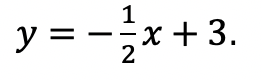## Perpendicular & Parallel Lines Through a Given Point: Geometry

Happy Wednesday math friends! Today we’re going to go over the difference between perpendicular and parallel lines, then we’ll use our knowledge of the equation of a line (y=mx+b) to see how to find perpendicular and parallel lines through a given point.  This is a common question that comes up on the NYS Geometry Regents and is something we should prepare for, so let’s go!

If you need any further explanation, don’t hesitate to check out the Youtube video below that goes into detail on how to find perpendicular and parallel lines through a given point one step at a time. Happy calculating! 🙂

## Perpendicular Lines:

Lines that intersect to create a 90-degree angle and can look something like the graph below.  Their slopes are negative reciprocals of each other which means they are flipped and negated. See below for example!

Example: Find an equation of a line that passes through the point (1,3) and is perpendicular to line y=2x+1 .

## Parallel Lines:

Parallel lines are lines that go in the same direction and have the same slope (but have different y-intercepts). Check out the example below!

Example: Find an equation of a line that goes through the point (-5,1) and is parallel to line y=4x+2.

Try the following practice questions on your own!

## Practice Questions:

1) Find an equation of a line that passes through the point (2,5) and is perpendicular to line y=2x+1.

2) Find an equation of a line that goes through the point (-2,4) and is perpendicular to line3)  Find an equation of a line that goes through the point (1,6) and is parallel to line y=3x+2.

4)  Find an equation of a line that goes through the point (-2,-2)  and is parallel to line y=2x+1.

## Solutions:

Need more of an explanation? Check out the video that goes over these types of questions up on Youtube (video at top of post) and let me know if you have still any questions.

Happy Calculating! 🙂

Looking for more on Perpendicular and parallel lines? Check out this Regents question on perpendicular lines here!